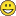New to QlikView

Discussion board where members can get started with QlikView.

Announcements
QlikWorld 2020: Join us May 11 - 14, 2020 in Phoenix, AZ. Register early and save \$400. Learn More
HighlightedPartner

Date Calculation and Cost Calculation Help

Hello:

I need to take an Approved_YearMonth value, add two months to it, then subtract 201801 to get the remaining number of months in 2017.  I then need to take the remaining number of months and multiply that by the Monthly Spend amount to get the 2017 \$\$\$ for that row when V, V-D, V-P, P or BES is greater than or less than zero.

Does is it make sense what I'm looking for?  I've attached some sample data which I hope will help.

As always, thanks in advance for any and all help.

Tags (1)
1 Solution

Accepted SolutionsMVP

Re: Date Calculation and Cost Calculation Help

The formula to calculate the Amount due is something like:

=(215 - (mid(Approved_YearMonth, 3, 2)*12+right(Approved_YearMonth, 2))) * [Monthly Spend]

The value 215 comes from an encoded number of months for date 201801. If we assume that your Dates never precede Jan 2000, then we drop the century and calculate 18 years * 12 months + 1. Then we subtract two months because you are reducing the delay by two months. Which results in 215 months. Using string manipulations we translate Approved_YearMonth using the same technique and calculate the number of remaining months.

I may be off by one month, because I'm not sure I understand why you add two months to the Approval date. For example, if Approved_YearMonth = 201710, then by adding 2 we get 201712 - 201801 = 1 month remaining. Is this correct?

Best,

Peter

6 RepliesMVP

Re: Date Calculation and Cost Calculation Help

The formula to calculate the Amount due is something like:

=(215 - (mid(Approved_YearMonth, 3, 2)*12+right(Approved_YearMonth, 2))) * [Monthly Spend]

The value 215 comes from an encoded number of months for date 201801. If we assume that your Dates never precede Jan 2000, then we drop the century and calculate 18 years * 12 months + 1. Then we subtract two months because you are reducing the delay by two months. Which results in 215 months. Using string manipulations we translate Approved_YearMonth using the same technique and calculate the number of remaining months.

I may be off by one month, because I'm not sure I understand why you add two months to the Approval date. For example, if Approved_YearMonth = 201710, then by adding 2 we get 201712 - 201801 = 1 month remaining. Is this correct?

Best,

PeterPartner

Re: Date Calculation and Cost Calculation Help

Hello Peter:

Thanks for the reply.  Quick question.  So for next year when I'm checking for how many months remain in 2018 (201901 - 2018xx), the 214 will become 227.  Is that right?

I will try the expression you provided in the next hour or so and let you know how it goes.Partner

Re: Date Calculation and Cost Calculation Help

I add two months because once an item is approved, it will take at least one month for it to be deployed which means we would not see a bill for it for two months.

For example, we approve the item in August, it gets deployed in September, so since it was not deployed in August, we do not receive a bill in September for August.  then, since it's deployed and "on the floor" in September, I will get a bill in October for September.

Think of it like your electric bill.  If you r billing period in 10/1 thru 10/31, you won't get a bill 10/15 for all of October because all October hasn't happened yet and thus, the electric company doesn't know what your consumption is / will be for 10/16 -  10/31, so you get your October bill in November.Partner

Re: Date Calculation and Cost Calculation Help

I tried the above expression (did you change 214 to 205????) and I'm getting primarily negative numbers.

If you look at the sample data, for DM # 45376, I have an Approved_YearMonth of 201708 and a Monthly Spend of \$223.  So, if I add 2 to 201708, it becomes 201710, then 201801 - 201710 is 3, so the 2017 \$\$\$ would be \$669.  Make sense?

Could it be that Approved_YearMonth is not formatted correctly?  I format it in my load script with the following:

Date(Date#([Approval Date], 'M/D/YYYY'), 'YYYYMM') as [Approved_YearMonth]Partner

Re: Date Calculation and Cost Calculation Help

Ok.  I get it.  The 205 should be 215 and then everything calculates as expected.  Can't tell you how many times I've fat fingered something.Thank you very much for the help!!!MVP

Re: Date Calculation and Cost Calculation Help

You're right Perry. I changed 215 into 205 before I left the office because I thought I made a mistake. I should have used a calculator instead of pen & paper.Corrected. Thanks for pointing out my mistake